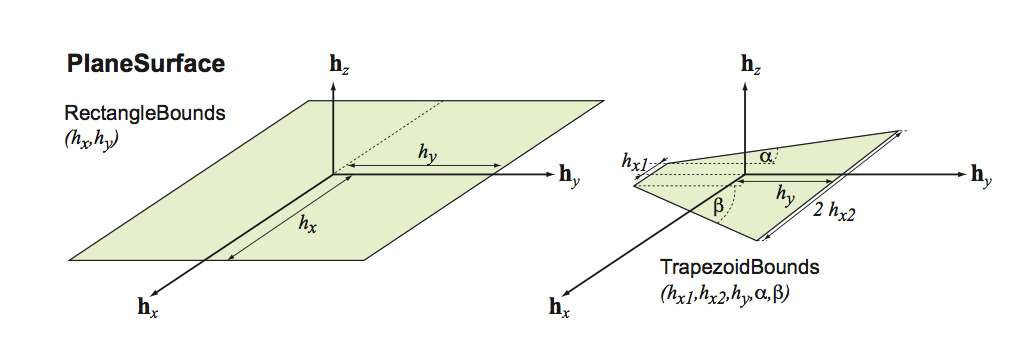# File PlaneSurface.hpp

namespace Acts

Note

This file is foreseen for the Geometry module to replace Extent

class PlaneSurface : public Acts::Surface
#include <Acts/Surfaces/PlaneSurface.hpp>

Class for a planaer in the TrackingGeometry.

The PlaneSurface extends the Surface class with the possibility to convert local to global positions (vice versa).Subclassed by Acts::PlaneLayer

Public Functions

PlaneSurface() = delete
~PlaneSurface() override = default
virtual Vector3 binningPosition(const GeometryContext &gctx, BinningValue bValue) const final

The binning position is the position calculated for a certain binning type.

Parameters
• gctx – The current geometry context object, e.g. alignment

• bValue – is the binning type to be used

Returns

position that can beused for this binning

virtual const SurfaceBounds &bounds() const override

Return method for bounds object of this surfrace.

virtual Result<Vector2> globalToLocal(const GeometryContext &gctx, const Vector3 &position, const Vector3 &direction, double tolerance = s_onSurfaceTolerance) const override

Global to local transformation.

Note

For planar surfaces the momentum direction is ignored in the global to local transformation

Parameters
• gctx – The current geometry context object, e.g. alignment

• position – global 3D position - considered to be on surface but not inside bounds (check is done)

• direction – global 3D momentum direction (optionally ignored) method symmetry)

• tolerance – optional tolerance within which a point is considered valid on surface

Returns

a Result<Vector2> which can be !ok() if the operation fails

virtual SurfaceMultiIntersection intersect(const GeometryContext &gctx, const Vector3 &position, const Vector3 &direction, const BoundaryCheck &bcheck = false, ActsScalar tolerance = s_onSurfaceTolerance) const final

Straight line intersection.

mathematical motivation:

the equation of the plane is given by: $$\vec n \cdot \vec x = \vec n \cdot \vec p,$$ where $$\vec n = (n_{x}, n_{y}, n_{z})$$ denotes the normal vector of the plane, $$\vec p = (p_{x}, p_{y}, p_{z})$$ one specific point on the plane and $$\vec x = (x,y,z)$$ all possible points on the plane.

Given a line with:$$\vec l(u) = \vec l_{1} + u \cdot \vec v$$

,

the solution for

$$u$$ can be written: $$u = \frac{\vec n (\vec p - \vec l_{1})}{\vec n \vec v}$$ If the denominator is 0 then the line lies:
• either in the plane

• perpendicular to the normal of the plane

Note

expected to be normalized)

Parameters
• gctx – The current geometry context object, e.g. alignment

• position – The start position of the intersection attempt

• direction – The direction of the intersection attempt, (

• bcheck – The boundary check directive

• tolerance – the tolerance used for the intersection

Returns

the SurfaceMultiIntersection object

virtual ActsMatrix<2, 3> localCartesianToBoundLocalDerivative(const GeometryContext &gctx, const Vector3 &position) const final

Calculate the derivative of bound track parameters local position w.r.t.

position in local 3D Cartesian coordinates

Parameters
• gctx – The current geometry context object, e.g. alignment

• position – The position of the parameters in global

Returns

Derivative of bound local position w.r.t. position in local 3D cartesian coordinates

virtual Vector3 localToGlobal(const GeometryContext &gctx, const Vector2 &lposition, const Vector3 &direction) const override

Local to global transformation.

Note

For planar surfaces the momentum direction is ignored in the local to global transformation

Parameters
• gctx – The current geometry context object, e.g. alignment

• lposition – local 2D position in specialized surface frame

• direction – global 3D momentum direction (optionally ignored)

Returns

the global position by value

virtual std::string name() const override

Return properly formatted class name for screen output.

inline Vector3 normal(const GeometryContext &gctx) const

Normal vector return without argument.

virtual Vector3 normal(const GeometryContext &gctx, const Vector2 &lposition) const final

Normal vector return.

return a Vector3 by value

Parameters
• gctx – The current geometry context object, e.g. alignment

• lposition – is the local position is ignored

Vector3 normal(const GeometryContext &gctx, const Vector2 &lposition) const = 0

Normal vector return without argument.

Vector3 normal(const GeometryContext &gctx, const Vector3 &position) const

Normal vector return without argument.

PlaneSurface &operator=(const PlaneSurface &other)

Assignment operator.

Parameters

other – The source PlaneSurface for assignment

virtual double pathCorrection(const GeometryContext &gctx, const Vector3 &position, const Vector3 &direction) const final

Method that calculates the correction due to incident angle.

Note

this is the final implementation of the pathCorrection function

Parameters
• gctx – The current geometry context object, e.g. alignment

• position – global 3D position - considered to be on surface but not inside bounds (check is done)

• direction – global 3D momentum direction (ignored for PlaneSurface)

Returns

a double representing the scaling factor

virtual Polyhedron polyhedronRepresentation(const GeometryContext &gctx, size_t lseg) const override

Return a Polyhedron for the surfaces.

Parameters
• gctx – The current geometry context object, e.g. alignment

• lseg – Number of segments along curved lines, it represents the full 2*M_PI coverange, if lseg is set to 1 only the extrema are given

Returns

A list of vertices and a face/facett description of it

virtual SurfaceType type() const override

Return the surface type.

Protected Functions

PlaneSurface(const GeometryContext &gctx, const PlaneSurface &other, const Transform3 &transform)

Copy constructor - with shift.

Parameters
• gctx – The current geometry context object, e.g. alignment

• other – is the source cone surface

• transform – is the additional transform applied after copying

PlaneSurface(const PlaneSurface &other)

Copy Constructor.

Parameters

other – is the source surface for the copy

PlaneSurface(const Transform3 &transform, std::shared_ptr<const PlanarBounds> pbounds = nullptr)

Constructor for Planes with (optional) shared bounds object.

Parameters
• transform – transform in 3D that positions this surface

• pbounds – bounds object to describe the actual surface area

PlaneSurface(const Vector3 &center, const Vector3 &normal)

Dedicated Constructor with normal vector This is for curvilinear surfaces which are by definition boundless.

Parameters
• center – is the center position of the surface

• normal – is thenormal vector of the plane surface

PlaneSurface(std::shared_ptr<const PlanarBounds> pbounds, const DetectorElementBase &detelement)

Constructor from DetectorElementBase : Element proxy.

Parameters
• pbounds – are the provided planar bounds

• detelement – is the linked detector element to this surface

Protected Attributes

std::shared_ptr<const PlanarBounds> m_bounds

the bounds of this surface